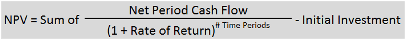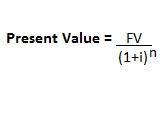# Accounting Equations & Formulas Flashcards

Accounting Equations & Formulas Flashcards
1/27 (missed) 0 0
Create Your Account To Continue Studying

As a member, you'll also get unlimited access to over 79,000 lessons in math, English, science, history, and more. Plus, get practice tests, quizzes, and personalized coaching to help you succeed.

Try it risk-free for 30 days. Cancel anytime
Owner's Equity Formula
Assets - Liability = Owner's equity
Got it
Cost of Capital
This represents the amount of money you lose by choosing not to make certain investments. For example, if you choose to lend money instead of investing it, you 'lose' money from interest.
Got it
Net Present Value FormulaGot it
Net Present Value (NPV)
We use this term when discussing how money's value shifts over the course of time.
Got it
Direct Costs
These costs are related clearly to the needs of a specific department or project in a business. They can include payroll, rent, travel costs and training expenses.
Got it
Cost of Goods Sold (COGS)
This refers to the cost necessary to create an item. It includes the cost of supplies, labor, utilities and packaging as well as the cost for space for the business and the equipment used.
Got it
Detailed Profit Formula
Total revenue - cost of goods sold = gross profit
Got it
Basic Profit Formula
Total revenue - explicit costs = basic profit
Got it
Opportunity Cost
Accountants use this term when referring to something that might have been a source of profit, but that was lost because a business chose to follow a different course.
Got it
Explicit Cost
These costs are easy for a company to identify. They can include wages, rent, taxes, payment for raw goods and more.
Got it
Income Statement
An accounting statement that details accounting profit and provides information about the expenses and revenue of a company. It's also referred to as a profit and loss statement.
Got it
Accounting Profit
This represents the revenue your company makes after taking out the costs of running the company. It's usually very close to your net income.
Got it
Accounting Profit Formula
Total revenue - (Cost of goods sold + operating expenses + taxes) = accounting profit
Got it

or choose a specific lesson: See all lessons in this chapter
27 cards in set

## Flashcard Content Overview

You can check out these flashcards to review the accounting equation, the extended accounting equation and the formulas to find prime cost, the future value of money, the present value of money and owner's equity. You'll also find cards that go over indirect costs, direct costs, cost of capital, cost of goods sold and prime cost. Additionally, the basic profit formula, the detailed profit formula and net present value will be covered.

Front
Back
Accounting Profit Formula
Total revenue - (Cost of goods sold + operating expenses + taxes) = accounting profit
Accounting Profit
This represents the revenue your company makes after taking out the costs of running the company. It's usually very close to your net income.
Income Statement
An accounting statement that details accounting profit and provides information about the expenses and revenue of a company. It's also referred to as a profit and loss statement.
Explicit Cost
These costs are easy for a company to identify. They can include wages, rent, taxes, payment for raw goods and more.
Opportunity Cost
Accountants use this term when referring to something that might have been a source of profit, but that was lost because a business chose to follow a different course.
Basic Profit Formula
Total revenue - explicit costs = basic profit
Detailed Profit Formula
Total revenue - cost of goods sold = gross profit
Cost of Goods Sold (COGS)
This refers to the cost necessary to create an item. It includes the cost of supplies, labor, utilities and packaging as well as the cost for space for the business and the equipment used.
Direct Costs
These costs are related clearly to the needs of a specific department or project in a business. They can include payroll, rent, travel costs and training expenses.
Net Present Value (NPV)
We use this term when discussing how money's value shifts over the course of time.
Net Present Value FormulaCost of Capital
This represents the amount of money you lose by choosing not to make certain investments. For example, if you choose to lend money instead of investing it, you 'lose' money from interest.
Owner's Equity Formula
Assets - Liability = Owner's equity
Owner's Equity
This represents the differences between the liabilities and assets of a company.
Return on Equity (ROE) Formula
Net income / shareholders' equity = return on equity
Present Value of Money FormulaBond
We use this term when referring to a promise made by a borrower to pay back the amount they borrowed in the future.
Present Value of Money
This refers to the amount of money it would take today to equal a series of future payments, each with an interest rate associated with them.
Future Value of Money
You can use this to discuss the worth in the future of a certain amount of money that has been invested and is earning a specific rate of interest.
Prime Cost Formula
Cost of raw materials + cost of direct labor (hours worked x wages) = Prime cost
Prime Cost
The total cost a business has to spend on labor and materials. Knowing this cost can allow a business to control costs of productions, which can increase a business's profits.
The Accounting Equation Formula
Formula Assets = liabilities + owner's equity
The Accounting Equation
An equation that you can use to demonstrate the relationship between liabilities, assets and an owner's equity.
Extended Accounting Equation Formula
Assets = liabilities + (revenue - (expenses + dividends))
Indirect Costs
These are the costs to a business that aren't directly associated with specific company projects. They can include utilities and general supplies to operate the business.
Future Value of Money Formula
PV * (1 + i)^n
Return on Equity (ROE) Ratio
This ratio tells you how much money you make for every dollar invested in your business. For example, if a business has 1.9 of this, then you make \$1.90 for every \$1 invested.

To unlock this flashcard set you must be a Study.com Member.